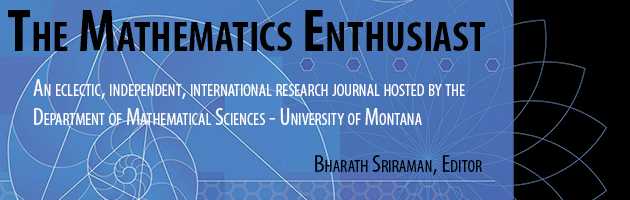•
•17

1

#### Abstract

A mathematical text is multimodal with different modes of communication, namely verbal language, algebraic notation, visual forms and gestures. This paper aims to compare and discuss the ideational meaning of visual forms in worked examples from Malaysian and Singaporean Grade Seven Mathematics textbooks on Lines and Angles. There are two structures in ideational meaning, namely narrative (with action) and conceptual (without action). Action diagrams represent ongoing mathematical activity whereas, without action diagrams represent mathematical objects. Document analysis and coding were carried on 57 geometrical diagrams found in the textbooks used in a 20-year period. The properties to identify a narratively and a conceptually structured diagram were based on grammar to ‘read’ geometrical diagrams. The Malaysian textbook used from the year 1997 to 2002 consisted of some narrative diagrams and the Singaporean textbooks consistently gave importance to conceptual diagrams. Further, there are differences in the classification, identifying and spatial relations between geometric elements among the series of textbooks and country. The geometrical diagrams in the Singaporean textbooks had given much importance to attributive letters compared with the Malaysian textbooks that had given much importance to letters to identify objects. Besides, the Singaporean textbooks had represented relations with ‘shapes’ whereas, the Malaysian textbooks had represented relations with ‘points’. The findings provide valuable information for educators in general to ‘read’ the ideational meaning of geometric diagrams and to construct better visual representations, especially in school textbooks.

166

199

#### Digital Object Identifier (DOI)

10.54870/1551-3440.1484

COinS